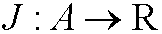# 14. A necessary condition for extremum of a functional.

Theorem 2: Let A  be a subset of a normed linear space V  and letbe a given functional. if an element y0 in A gives a local minimum for J  relative the norm ||.|| thenRemark: The solutions to the problem above is called extremals.

Proof: Define the functionThis obviously a regular one-variable functionand a necessary condition for extreme values to be attained in the pointis thatthat is,The proof is complete.